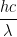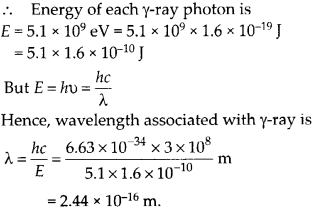Enlightened

# Question 24: NCERT Solutions for 12th Class Physics: Chapter 11-Dual Nature of Radiation and Matter

• 0

Question 24: NCERT Solutions for 12th Class Physics: Chapter 11-Dual Nature of Radiation and Matter

In an accelerator experiment on high energy collisions of electrons with positrons, a certain event is interpreted as annihilation of an electron positron pair of total energy 10.2 BeV into two y-rays of equal energy. What is the wavelength associated with each y-ray? (1 BeV = 109 eV).

Share

1. Solution:
In the process of annihilation, the total energy of electron-positron pair is shared equally by both y ray photons produced. Energy of two y-rays = Energy of electron- positron pair = 10.2 BeV = 10.2 × 109 eV Energy of each y-ray photon is E = 5.1 × 109 eV = 5.1 x 109 x 1.6 x 10-19 J = 5.1 × 1.6 x 10-10 J But E = hv =Hence, wavelength associated with y-ray isCheck the complete chapter with solutions.
NCERT Solutions for 12th Class Physics: Chapter 11-Dual Nature of Radiation and Matter

• 0